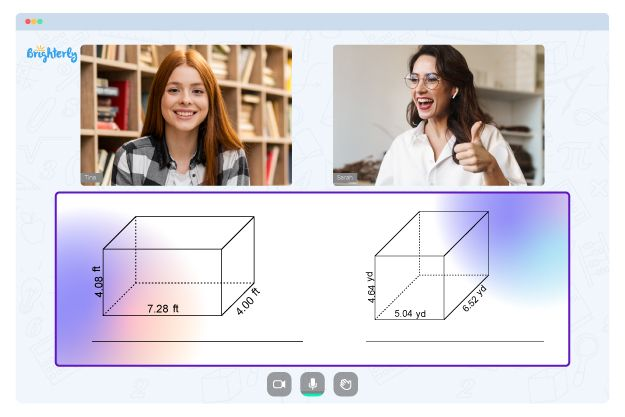# Similar Triangles Worksheets

Ever heard of similar triangles? As kids climb up the academic ladder, they’re introduced to a wide range of mathematical concepts, including basic geometry. To a large extent, geometry is fun. However, keeping up with the different types of shapes can be a tad overwhelming for any learner.

Similar triangles are an essential part of geometry that kids have to learn before moving on to a new concept. Unfortunately, it comes with a deluge of questions that need clarity, such as “What are similar triangles?” and “Are they the same as congruent triangles?”

On the flip side, a similar triangles worksheet helps to clear the air and teaches kids all they need to know about this topic. Let’s get into it, shall we?

## What Are Similar Triangles?

More often than not, learners mix up similar triangles with congruent ones. However, an entire world of difference exists between these two concepts.

Congruent triangles are triangles that have both the same shape and the same size. On the other hand, similar triangles have the same shape but may not have the same size. The sizes of similar triangles typically vary.

To ascertain the similarity of two triangles, you’ll need to check if they meet one of the following criteria:

• Three pairs of corresponding sides are proportional.
• Two pairs of corresponding angles are equal.
• Two pairs of corresponding sides are proportional, and the corresponding angles between them are equal.

Math for Kids

Is Your Child Struggling With Math?
1:1 Online Math TutoringThe triangle similarity worksheet is designed to help kids master this mathematical concept. It features a wide range of exercises covering trigonometric theorems and expressions.

After using this worksheet, kids will learn how to identify similar triangles and determine the scale factor of said triangles. They’ll also learn how to calculate the side lengths of triangles and find similarities based on SSS, SAS, and AA theorems.

The exercises contained within a proving similar triangles worksheet are arranged progressively, ranging from easy to difficult tasks. This approach helps young learners get acclimatized to the concept without being thrown head-on into complex exercises. As time goes on, they’ll become pros at identifying similar triangles.### Similar Triangles Worksheets PDF

Similar Triangles Worksheet### Similar Triangles Worksheets PDF### Similar Triangles Worksheets PDF

Proving Similar Triangles Worksheet### Similar Triangles Worksheets PDF

Triangle Similarity Worksheet

In addition, at the end of the worksheet, you can find answers to all the exercises. The similar triangles worksheet answers help young learners confirm that they’re on the right track. The answers are also an excellent hack for self-guided or self-led learning sessions.

## Benefits of the Similar Triangle Worksheet Answer Key

The similar triangles worksheet provides learners with an accurate understanding of the concept. As they go through the exercises, they’ll be able to identify similar triangles faster and with more accuracy. Over time, they’ll develop strong problem-solving and analytical skills that can be transferred to other math concepts and topics.

### More Worksheets

Struggling with Geometry?• Start lessons with an online tutor.

Is your child finding it challenging to master of geometry? An online tutor could be the solution.